# Controlled Stochastic Process

The following article is from The Great Soviet Encyclopedia (1979). It might be outdated or ideologically biased.

## Controlled Stochastic Process

a stochastic process whose probability characteristics can be changed by means of control actions. The main goal of the theory of stochastic control is to find optimal or near-optimal controls that provide an extremum for a given performance criterion.

Let us take the simple case of controlled Markov chains and consider one of the ways in which a mathematical statement of the problem of finding the optimal control can be formulated. Suppose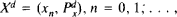is a family of homogeneous Markov chains with a finite number of states E = {0,1,..., N} and matrices of transition probabilities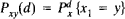. The transition probabilities depend on the parameter d, which belongs to some set of control actions D. The set of functions α = {α0(x0), α1(x0, x1,...} with values in D is called the strategy, and each of the functions αn = αn (x0, ..., xn) is called the control at time n. To every strategy α there corresponds a controlled Markov chain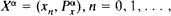where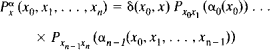Let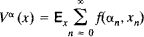where the function f(d, x) ≥ 0 and f(d, 0) = 0. (If the point {0} is an absorbing state and f(d, x) = 1, dD, x = 1, . . ., N, then Vα(x) is the mathematical expectation of the time of transition from point x to point 0.) The function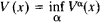is called the value, and the strategy α* is said to be optimal if

Vα* (x) = V (x)

for all xE.

Under quite general assumptions regarding the set D, it can be shown that the value V(x) satisfies the following optimality equation (the Bellman equation):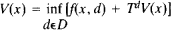where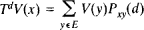In the class of all strategies, homogeneous Markovian strategies, which are characterized by a single function α(x) such that αn (x0,...,xn) = α(xn) for all n = 0, 1, ..., are of the greatest interest.

The following optimality criterion, or sufficient condition for optimality, can be used to verify that a given homogeneous Markovian strategy is optimal: let there be functions α* = α*(x) and V* = V*(x) such that for any dD

0 = f(x, α*(x)) + Lα* V* ≤ f(x, d) + LdV*(x)

(where Ld = TdI, I being the identity operator), then V* is the value (V* = V), and the strategy α* = α*(x) is optimal.

### REFERENCE

Howard, R. A. Dinamicheskoe programmirovanie i markovskie protsessy. Moscow, 1964. (Translated from English.)

A. N. SHIRIAEV